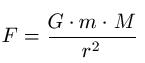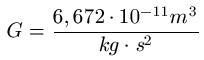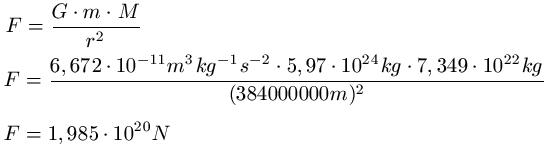# What is the example of gravitational force

## Gravitational force

We will deal with the force of gravity in this article. It explains what is meant by gravitational force and how to calculate with it. This article belongs to the field of physics / mechanics.The law of gravity was discovered by Isaac Newton in the 17th century. With this, the so-called gravitational force can be calculated. The force that two bodies exert on each other at a certain distance can be calculated. The formula for calculating the gravitational force is:Where:

• "F" The force between the two masses in Newtons [N]
• "G" The gravitational constant [m3 kg-1 s-2 ]
• "m" The mass of the first mass point in kilograms [kg]
• "M" The mass of the second mass point in kilograms [kg]
• "r" The distance between the mass points in meters [m]

Note: The gravitational constant isExample of gravitational force:

The mass of the earth is roughly 5.97 x 1024 kg, the mass of the moon about 7.349 · 1022 kg. The distance between the earth and the moon is constantly changing. For the calculation we use 384,000 km. How big is the gravitational force between the earth and the moon?Left:

### Who's Online

We have 320 guests online